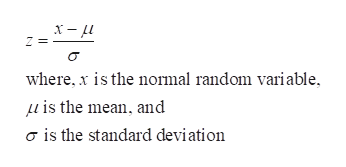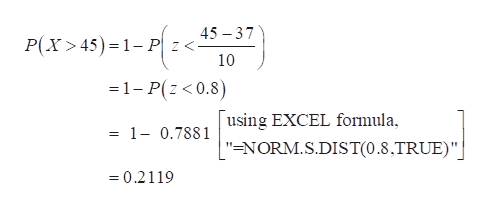# The percent of fat calories that a person in America consumes each day is normally distributed with a mean of about 37 and a standard deviation of 10. Suppose that one individual is randomly chosen. Let X=percent of fat calories.a. What is the distribution of X? X ~ N(,)b. Find the probability that a randomly selected fat calorie percent is more than 45. Round to 4 decimal places.c. Find the minimum number for the lower quartile of fat calories. Round to 2 decimal places.

Question
8 views

The percent of fat calories that a person in America consumes each day is normally distributed with a mean of about 37 and a standard deviation of 10. Suppose that one individual is randomly chosen. Let X=percent of fat calories.

a. What is the distribution of X? X ~ N(,)

b. Find the probability that a randomly selected fat calorie percent is more than 45. Round to 4 decimal places.

c. Find the minimum number for the lower quartile of fat calories. Round to 2 decimal places.

check_circle

Step 1

Standard normal distribution:

The standard normal distribution is a special case of normal distribution, in which the mean of the distribution is 0 and standard deviation of the distribution is 1. That is X~N(µ, σ). The Z-scores can be calculated using the given formulahelp_outlineImage Transcriptionclosewhere, x is the normal random variable is the mean, and o is the standard deviation fullscreen
Step 2

1.

In this context, it is given that the average percent of fat calorie  is 37 corresponding to its standard deviation of 10.

Denote X as the percent of fat calorie.

In this X follows normal with mean of 37 and standard deviation of 10. That is X~N(37, 10).

Step 3

2.

The probability that a randomly selected fat calori...help_outlineImage Transcriptionclose45 37 P(X 45 1 P Z<- 10 1- P(z <0.8) using EXCEL formula, 1- 0.7881 = "=NORM.S.DIST(0.8,TRUE)" =0.2119 fullscreen

### Want to see the full answer?

See Solution

#### Want to see this answer and more?

Solutions are written by subject experts who are available 24/7. Questions are typically answered within 1 hour.*

See Solution
*Response times may vary by subject and question.
Tagged in
MathStatistics

### Hypothesis Testing